### DIFFERENTIATION OF TRIGONOMETRY FUNCTIONS

In the following discussion and solutions the derivative of a function h(x) will be denoted by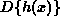or h'(x) . The following problems require the use of these six basic trigonometry derivatives :
1.2.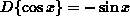3.4.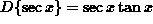5.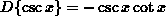6.These rules follow from the limit definition of derivative, special limits, trigonometry identities, or the quotient rule. In the list of problems which follows, most problems are average and a few are somewhat challenging.

On problems 1.) through 8.) find answers WITHOUT using the chain rule.

• PROBLEM 1 : Differentiate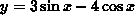.

• PROBLEM 2 : Differentiate.

• PROBLEM 3 : Differentiate.

• PROBLEM 4 : Differentiate.

• PROBLEM 5 : Differentiate.

• PROBLEM 6 : Differentiate.

• PROBLEM 7 : Differentiate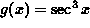.

• PROBLEM 8 : Evaluate.

Some of the following problems require use of the chain rule.

• PROBLEM 9 : Differentiate.

• PROBLEM 10 : Differentiate.

• PROBLEM 11 : Differentiate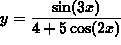.

• PROBLEM 12 : Differentiate.

• PROBLEM 13 : Differentiate.

• PROBLEM 14 : Differentiate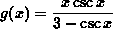.

• PROBLEM 15 : Find an equation of the line tangent to the graph ofat x=-1 .

• PROBLEM 16 : Find an equation of the line perpendicular to the graph of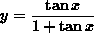at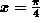.

• PROBLEM 17 : Assume that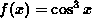. Solve f'(x) = 0 for x in the interval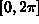.

• PROBLEM 18 : Use any method to verify that.